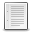File : Details

# Matrix and vector generator for Chebyshev Polynomials of the First Kind

Generators of Chebyshev Polynomials of the First Kind and its 1st &2nd derivative matrices
Details
Version
1.0
Author
Brenton Horne
Maintainer
Brenton Horne
Category
Supported Scilab Version
5.4
Creation Date
February 20, 2012Description
```            	A simple Scilab file that calculates the matrices with components T_n (x_m),
T'_n(x_m), T''_n(x_m) and calcualtes the extrema grid points.            ```Files (1)Miscellaneous file
```	A simple Scilab file that calculates the matrices with components T_n (x_m),
T'_n(x_m), T''_n(x_m) and calcualtes the extrema grid points.
```News (0)Comments (0)